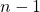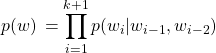# What order of Markov assumption does n-grams model make ?

An n-grams model makes order n-1 Markov assumption. This assumption implies: given the previous n-1 words, probability ofword is independent of words prior towords.

Suppose we have k words in a sentence, their joint probability can be expressed as follows using chain rule:Now, the Markov assumption can be used to make the above factorization simpler, where each word in a sequence depends only on the previous n-1 words for an n grams model.

For bi-gram model(n=2), first order Markov assumption is made and the above expression becomesFor tri-gram model(n=3), second order Markov assumption is made, which means probability of a word depends on previous 2 words, hence second order.Thinking exercise – how do you handle words like?